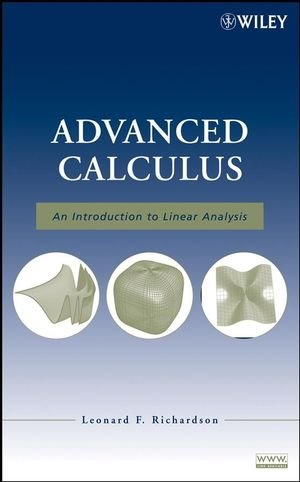# Advanced Calculus: An Introduction to Linear Analysis

• Length: 416 pages
• Edition: 1
• Publisher:
• Publication Date: 2008-04-25
• ISBN-10: 0470232889
• ISBN-13: 9780470232880
Description

Features an introduction to advanced calculus and highlights its inherent concepts from linear algebra

Advanced Calculus reflects the unifying role of linear algebra in an effort to smooth readers’ transition to advanced mathematics. The book fosters the development of complete theorem-proving skills through abundant exercises while also promoting a sound approach to the study. The traditional theorems of elementary differential and integral calculus are rigorously established, presenting the foundations of calculus in a way that reorients thinking toward modern analysis.

Following an introduction dedicated to writing proofs, the book is divided into three parts:

Part One explores foundational one-variable calculus topics from the viewpoint of linear spaces, norms, completeness, and linear functionals.

Part Two covers Fourier series and Stieltjes integration, which are advanced one-variable topics.

Part Three is dedicated to multivariable advanced calculus, including inverse and implicit function theorems and Jacobian theorems for multiple integrals.

Numerous exercises guide readers through the creation of their own proofs, and they also put newly learned methods into practice. In addition, a “Test Yourself” section at the end of each chapter consists of short questions that reinforce the understanding of basic concepts and theorems. The answers to these questions and other selected exercises can be found at the end of the book along with an appendix that outlines key terms and symbols from set theory.

Guiding readers from the study of the topology of the real line to the beginning theorems and concepts of graduate analysis, Advanced Calculus is an ideal text for courses in advanced calculus and introductory analysis at the upper-undergraduate and beginning-graduate levels. It also serves as a valuable reference for engineers, scientists, and mathematicians.